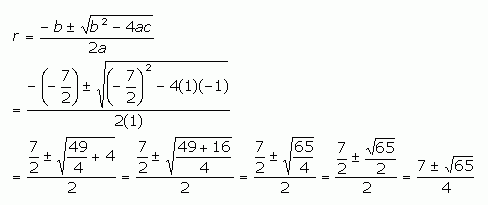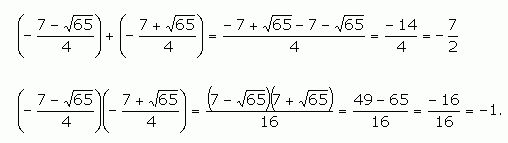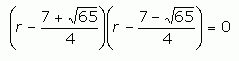SEARCH HOMEMath Central Quandaries & QueriesQuestion from Aranxa, a student: how do u solve this equation by factoring: 10r^2 - 35r = 10Hi Aranxa.

I would first make an algebraic expression equal to zero by subtracting 10 from both sides.

This gives: 10r2 - 35r - 10 = 0. Now you can divide both sides by 10, so

r2 - 7/2 r - 1 = 0.

To factor this quadratic expression, you need to find two numbers that when added give -7/2 and when multiplied give -1. In this case, the factors are irrational and very hard to conjure up mentally.

For such cases, we turn to the Quadratic Formula instead.

Thus,So if r equals this, then that means that when we add these values, we get -7/2 and when we multiply we should get -1. Let's test this:Yes, both these check out. So we can write the factorization as:But I could never come up with this by mentally factoring it: the quadratic formula is needed.

Stephen La Rocque .Math Central is supported by the University of Regina and The Pacific Institute for the Mathematical Sciences.